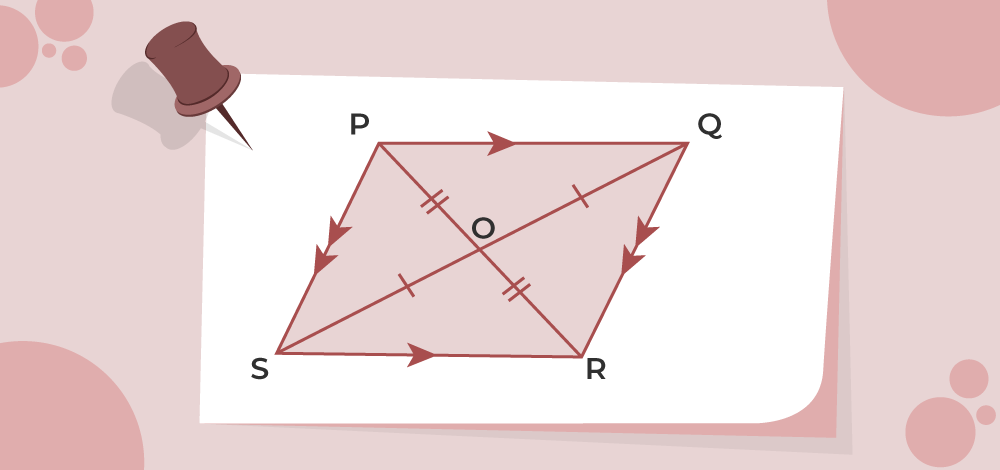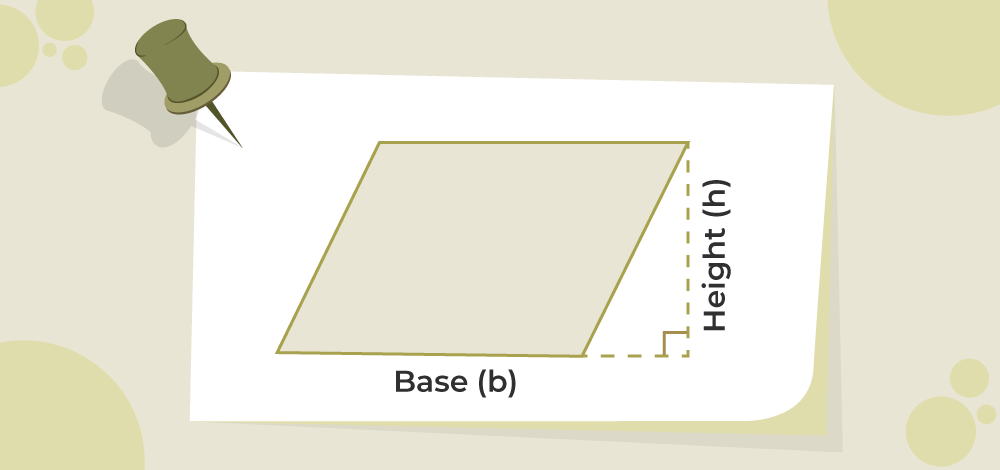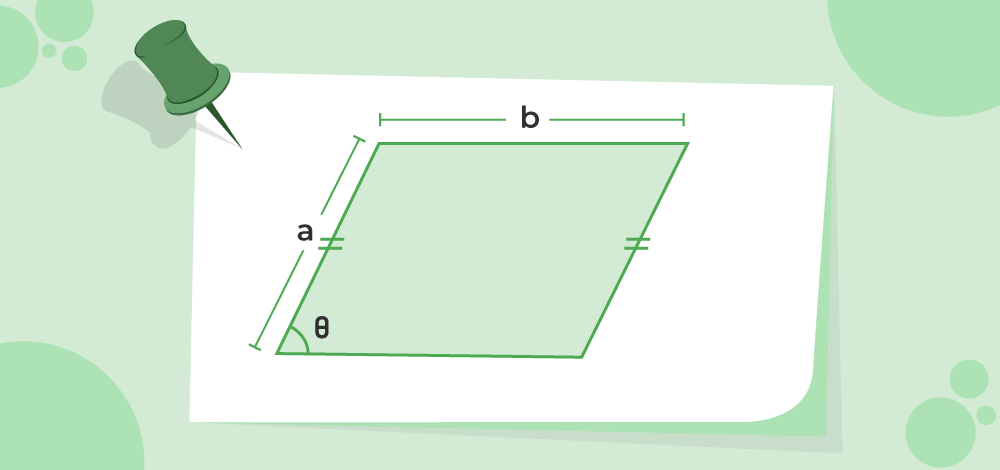GFG App
Open AppBrowser
Continue

Area of a parallelogram is the space or the region enclosed by the boundary of the parallelogram in a two-dimensional space. It is defined as the area covered inside the perimeter of the parallelogram. It is calculated by multiplying the base of the parallelogram by its height. It is calculated in unit square and the standard unit for the measurement of the area of the parallelogram is m2.

## What is the Area of a Parallelogram?

The total surface occupies by the parallelogram on a two-dimensional plane surface is the area of a parallelogram. The area of a parallelogram can be determined by using its height, length of sides, or diagonals. As the area of the parallelogram is nothing but the area it is measured in the unit square, i.e. m2, cm2## Area of a Parallelogram Formula

The area of a parallelogram can be determined by multiplying its base by its altitude. As seen in the following diagram, a parallelogram’s base and height are perpendicular to one another. Thus, the following formula can be used to determine a parallelogram’s area,

Area of Parallelogram = Base × Height

A = b × h

where,
b is the base of Parallelogram
h is the height of Parallelogram## How to Find the Area of a Parallelogram?

Generally, the area of a parallelogram can be calculated using its base and height. In addition to that, the area of a parallelogram can also be determined if the lengths of its parallel sides and any angles between them are known, as well as the angles at which its two diagonals intersect. As a result, there are three ways to calculate the parallelogram’s area:

• When the Base and Height of the parallelogram are known.
• When the lengths of the sides of the parallelogram are known.
• When the lengths of the diagonals of the parallelogram are known.

### Area of a Parallelogram using Base and Height

The area of a parallelogram using the height is given by the product of its base and height.

Mathematically it is written as

Area of parallelogram = b × h

where,
b is the base of Parallelogram
h is the height of Parallelogram

Example: Find the area of a parallelogram whose base is 12 cm and height is 8 cm.

Solution:

Given that,

Base (b) = 12 cm

Height (h) = 8 cm

The formula to calculate the area of a parallelogram is,

A = b × h

A = 12 × 8

A = 96 cm2

### Area of a Parallelogram using Side Lengths

The area of a parallelogram can be calculated by using the length of sides and adjacent angles if the height is not given.

Mathematically it is written as,

Area of Parallelogram = ab sin (θ)

where,
a and b are the lengths of parallel sides
θ is the angle between the sidesExample: If the angle between two sides of a parallelogram is 30 degrees and the length of its adjacent sides are 5 cm and 6 cm. Determine the area of the parallelogram.

Solution:

Given that,

Length of one side (a) = 5 cm,

Length of the other side (b) = 4 cm,

Angle between the two adjacent sides (θ) = 30 degrees

The formula to calculate the area of a parallelogram is,

A = ab sin (θ)

A = 5 × 4 × sin (30)

A = 10 cm2

### Area of a Parallelogram using Diagonals

A parallelogram consists of two diagonals that intersect each other at a certain angle meeting at a particular point. The area of a parallelogram can be calculated by using the length of its diagonals.

The formula for the area of a parallelogram by using the length of diagonals is given by,

Area of parallelogram = 1/2 × d1 × d2 sin (x)

where,
d1 and d2 are the lengths of the diagonals
x is the angle between the diagonalsExample: Determine the area of the parallelogram, when the angle between two intersecting diagonals of a parallelogram is 90 degrees and the length of its diagonals are 2 cm and 6 cm.

Solution:

Given that,

Length of one diagonal (d1) = 2 cm,

Length of the other diagonal (d2) = 6 cm,

Angle between the two intersecting diagonals (x) = 90 degrees

The formula to calculate the area of a parallelogram is,

A = 1/2 × d1 × d2 sin (x)

A = 1/2 × 2 × 6 × sin (90)

A = 6 cm2

## Area of Parallelogram Vector

The area of a parallelogram can be calculated even when the sides and the diagonals of the parallelogram are given in vector form. Considering a parallelogram PQRS, with adjacent sidesandrespectively. And the diagonals areand.

Now, the area of the parallelogram in vector form is given by using the adjacent sidesandas,Using the Parallelogram Law of Vector AdditionNow,But,andTherefore,Thus from equation (1), the area of the parallelogram in vector form is stated as:## Summary

• Area of a parallelogram using Base and Height,

A = b × h

• Area of a parallelogram using the side lengths,

A = ab sin (θ)

• Area of parallelogram using diagonals,

A = 1/2 × d1 × d2 sin (x)

## Solved Examples on Area of a Parallelogram

Example 1: Find the area of a parallelogram whose base is 10 cm and height is 8 cm.

Solution:

Given,

Base (b) = 10 cm

Height (h) = 8 cm

We have,

A = b × h

= 10 × 8

= 80 cm2

Example 2: Find the area of a parallelogram whose base is 5 cm and height is 4 cm.

Solution:

Given,

Base (b) = 5cm

Height (h) = 4cm

Area(A) = b × h

A = 5 × 4

= 20 cm2

Example 3: Determine the area of the parallelogram, when the angle between two intersecting diagonals of a parallelogram is 90 degrees and the length of its adjacent sides are 4 cm and 8 cm.

Solution:

Given that,

Length of one diagonal (d1) = 4 cm,

Length of the other diagonal (d2) = 8 cm,

Angle between the two intersecting diagonals (x) = 90 degrees

The formula to calculate the area of a parallelogram is,

A = 1/2 × d1 × d2 sin (x)

A = 1/2 × 4 × 8 × sin (90)

A = 16 cm2

Example 4: If the angle between two sides of a parallelogram is 60 degrees and the length of its adjacent sides is 3 cm and 6 cm. Determine the area of the parallelogram.

Solution:

Given that,

Length of one side (a) = 3 cm,

Length of the other side (b) = 6 cm,

Angle between the two adjacent sides (θ) = 60 degrees

The formula to calculate the area of a parallelogram is,

A = ab sin (θ)

A = 3 × 6 × sin (60)

A = 15.6 cm2

Example 5: Find the area of a parallelogram whose parallel sides are 4 cm and 3 cm and the angle between these sides is 90°.

Solution:

Given,

Let the lengths of the sides by a and b with values 4 cm and 3 cm respectively.

Angle between the sides 90°

Area = ab sinθ

A = 4 × 3 sin 90°

A = 12 cm2

## FAQs on Area of a Parallelogram

### Q1: What is the Area of a Parallelogram?

Area of parallelogram is the area inclosed by the  boundary of the parallelogram it can be defined as the 2-D space inside the perimeter of the parallelogram.

### Q2: What is the Perimeter of a Parallelogram?

Perimeter of a parallelogram is defined as the sum of its all four sides, so is given as,

P = 2 (a + b)

where
a and b are the length of the opposite sides of a parallelogram

### Q3: What are the Properties of a Parallelogram?

The Properties of Parallelogram are,

• Opposite sides of a parallelogram are equal and parallel to each other.
• Opposite angles of a parallelogram are equal.
• Sum of interior angles of a parallelogram is equal to 360°.
• Adjacent angles of a parallelogram must be supplementary i.e. equal to 180°.

### Q4: What is the formula for finding the height of a parallelogram?

The formula for the height of a parallelogram, when the area and the base of a parallelogram are known then:

h = A/b

where,
h is the height
b is the base
A is the area of the parallelogram

### Q5: How to calculate the area of a parallelogram?

Area of paralleogram is calculated using the formula,

Area = B × H

where,
B is the base of the parallelogram
H is the height of the parallelogram

### Q6: How to find the area of a parallelogram without height?

The area of a parallelogram when height is not given is calculated using the formula,

Area = ab sin (θ)

where,
a and b are the lengths of parallel sides
θ is the angle between the sides.

### Q7: How to find the area of parallelogram when diagonals are given?

The area of a parallelogram when diagonals are given is calculated using the formula,

Area = 1/2 × d1 × d2 sin (x)

where,
d1 and d2 are the lengths of the diagonals
x is the angle between the diagonals